• 对数回归分析
2021-04-19 01:45:38

公式推导

对数几率回归用于处理二分类问题，其数学基础为对数几率函数，是一种 Sigmoid 函数

$y = \frac{1}{1+e^{-z}} \tag 1$

其函数图像如下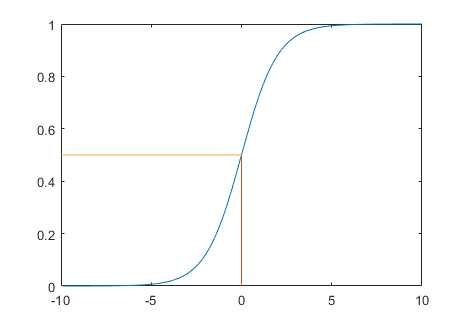取 $z = \boldsymbol{w}^T\boldsymbol{x}+b$，并对式 $(1)$ 进行一定变换，得

$$\ln\frac{y}{1-y}= \boldsymbol{w}^T\boldsymbol{x}+b \tag 2\$$

可以理解为，使用线性回归的预测结果逼近真实标记的对数几率. 当 $y>0.5$ 时，左式大于 $0$；当 $y<0.5$ 时，左式小于$0$.

按照线性回归模型的求解过程，推导出求解 $$\boldsymbol w$$ 和 $$b$$ 的迭代公式. 将式 $$(2)$$ 中的 $$y$$ 看作类后验概率估计$$p(y=1|\boldsymbol x)$$, 代入式 $$(2)$$ 可以推出

\begin{align} p(y=1|\boldsymbol x) = \frac{e^{\boldsymbol w^T\boldsymbol x+b}}{1+e^{\boldsymbol w^T \boldsymbol x+b}} \tag 3\p(y=0|\boldsymbol x) = \frac{1}{1+e^{\boldsymbol w^T \boldsymbol x+b}} \tag 4\\end{align}

令 $$\hat{\boldsymbol w} = (\boldsymbol w;b), \hat{\boldsymbol x_i}=(\boldsymbol x_i;1)$$ 采用极大似然估计法估计 $$\hat{\boldsymbol w}$$ ,最大化函数

$L(\hat{\boldsymbol w}) = \sum\limits_{i=1}^m\ln p(y_i|\hat{\boldsymbol x_i},\hat{\boldsymbol w}) \tag 5\$

令$$p_1(\hat{\boldsymbol x_i},\hat{\boldsymbol w}) = p(y=1|\hat{\boldsymbol x_i},\hat{\boldsymbol w}), p_0 = 1-p_1(\hat{\boldsymbol x_i},\hat{\boldsymbol w})$$，代入式 $$(5)$$，得

$L(\hat{\boldsymbol w}) = \sum\limits_{i=1}^m\ln (y_ip_1(\hat{\boldsymbol x_i},\hat{\boldsymbol w})+(1-y_i)p_0(\hat{\boldsymbol x_i},\hat{\boldsymbol w})) \tag 6\$

代入式 $$(3)(4)$$，最大化式 $$(6)$$ 等价于最小化

$L(\hat{\boldsymbol w}) = \sum\limits_{i=1}^m (-y_i\hat{\boldsymbol w}^T\hat{\boldsymbol x_i}+\ln(1+e^{\hat{\boldsymbol w}^T\hat{\boldsymbol x_i}}))\tag 7\$

使用牛顿迭代法得到迭代更新公式

\begin{align} \hat{\boldsymbol w} &\leftarrow \hat{\boldsymbol w}-\left(\frac{\partial^2L(\hat{\boldsymbol w})}{\partial \hat{\boldsymbol w} \partial\hat{\boldsymbol w}^T} \right )^{-1}\frac{\partial L(\hat{\boldsymbol w})}{\partial\hat{\boldsymbol w}} \tag 8\\frac{\partial L(\hat{\boldsymbol w})}{\partial\hat{\boldsymbol w}} &=-\sum\limits_{i=1}^m \hat{\boldsymbol x_i}(y_i-p_1(\hat{\boldsymbol x_i},\hat{\boldsymbol w})) \tag 9\\frac{\partial^2L(\hat{\boldsymbol w})}{\partial \hat{\boldsymbol w} \partial\hat{\boldsymbol w}^T} &=\sum\limits_{i=1}^m\hat{\boldsymbol x_i}\hat{\boldsymbol x_i}^Tp_1(\hat{\boldsymbol x_i},\hat{\boldsymbol w})(1-p_1(\hat{\boldsymbol x_i},\hat{\boldsymbol w})) \tag {10} \end{align}

其中式 $$(9)$$ 可以向量化为

$\frac{\partial L(\hat{\boldsymbol w})}{\partial\hat{\boldsymbol w}} = \boldsymbol X^T(p_1({\boldsymbol X},\hat{\boldsymbol w})-\boldsymbol y) \tag{11}$

MATLAB 实现

% 生成随机训练样本，直线 y=0.7x+200 上方的为正例，下方为反例

% 输出训练样本在坐标轴上的分布

x = zeros(100, 2);

y = zeros(100, 1);

kb = [0.7,200];

figure;

hold on;

for i = 1:100

x(i,1) = randi(1000,1);

x(i,2) = randi(1000,1);

if kb(1)*x(i,1)+kb(2)>x(i,2)

plot(x(i,1), x(i,2), ‘r*‘);

y(i) = 1;

else

plot(x(i,1),x(i,2), ‘b*‘);

y(i) = 0;

end

end

% 牛顿迭代法解权重

function w = cal(X,y,eps)

[m,n] = size(X);

X = [X ones(m,1)];

n = n + 1;

w = zeros(n, 1);

w(3) = 1000;

prew = zeros(n, 1);

while (true)

flag = 0;

sum1 = X‘*(1-1./(1+exp(X*prew))-y);

sum2 = 0;

for i = 1:m

sum2 = sum2 + X(i,:)*X(i,:)‘*(1-1./(1+exp(X(i,:)*prew)))*(1./(1+exp(X(i,:)*prew)));

end

w = prew - sum1./sum2;

for i = 1:n

if abs(w(i)-prew(i))>eps

flag = 1;

end

end

if flag==0

break;

end

prew = w;

end

end

% 测试并输出训练效果图像

eps = 0.0001;

w = cal(x, y, eps);

figure;

hold on;

for i=1:100

yy = w(1)*x(i,1)+w(2)*x(i,2)+w(3);

if yy>0

plot(x(i,1), x(i,2), ‘r*‘);

else

plot(x(i,1), x(i,2), ‘b*‘);

end

end

训练集的真实分布(红色正例、蓝色反例)：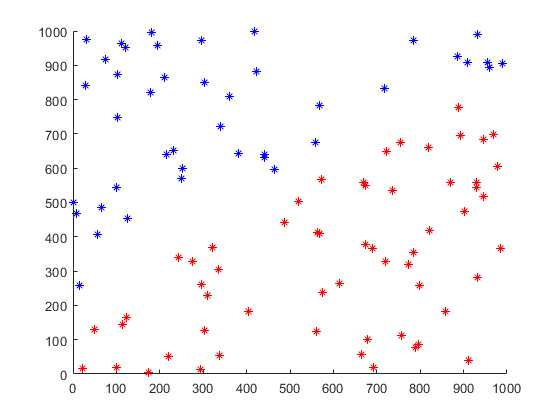模型的分类效果：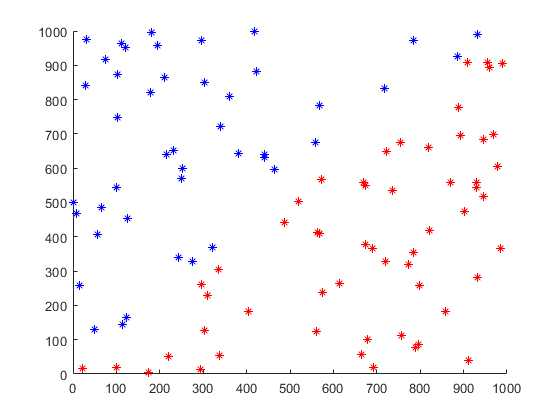原文：https://www.cnblogs.com/Kusunoki/p/13817347.html

更多相关内容
• 背景：很多业务并不是线性增长，当增长某一程度时，其增长率会开始减缓1、准备数据 案例中准备数据如下 以excel2013为例选择数据“插入”散点图，见下图选择增加“趋势线”选择对数配置，选择公式显示，便可以看到...

背景：很多业务并不是线性增长，当增长某一程度时，其增长率会开始减缓

1、准备数据

案例中准备数据如下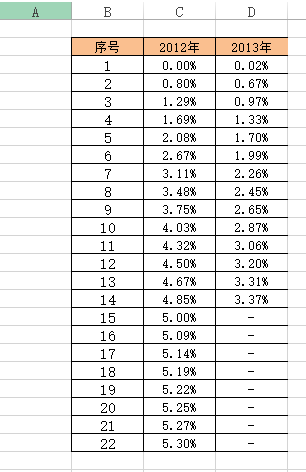以excel2013为例

选择数据“插入”散点图，见下图选择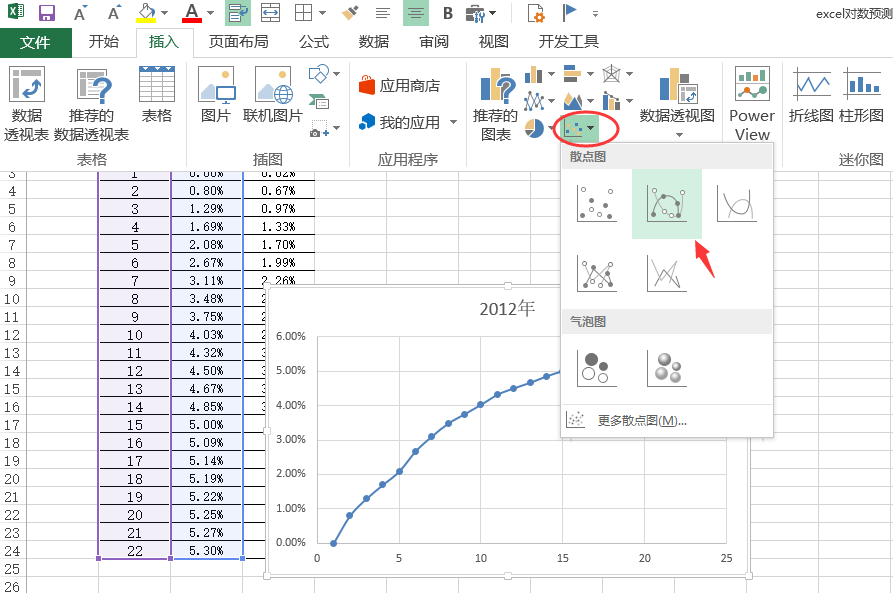增加“趋势线”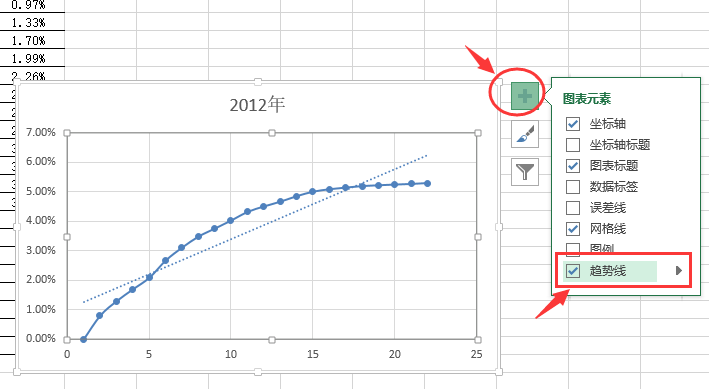选择对数配置，选择公式显示，便可以看到公式
也可以向前或后预测，图中实例向后预测5期，结果显示如下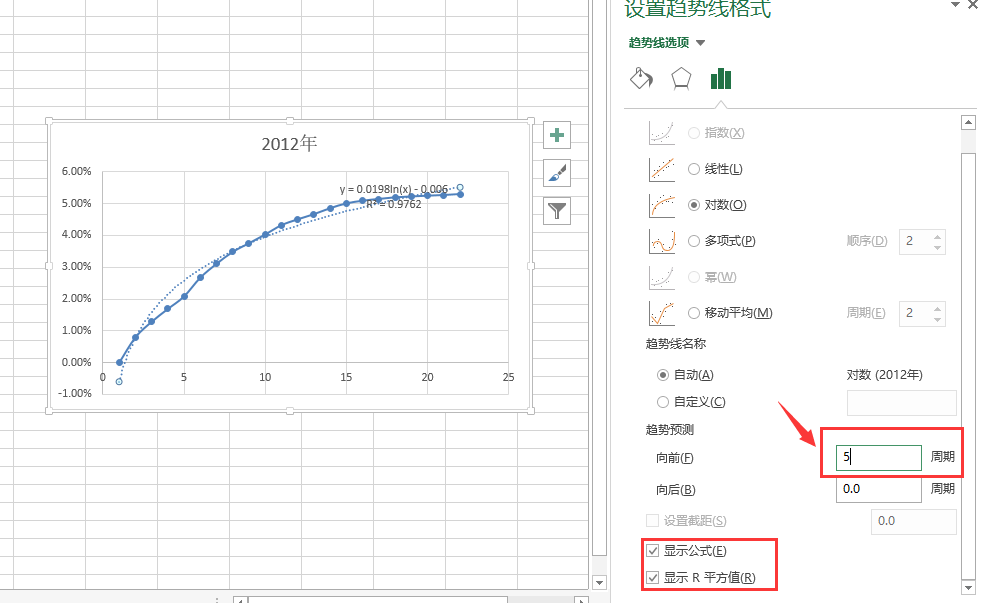展开全文• 1 对数几率回归原理分析 1.1 引入 1.2 损失函数 1.3 求最优解 2 对数几率回归实践 Logistic回归的一般过程 Logistic回归的优缺点 Logistic回归算法描述（改进的随机梯度下降） 《机器学习实战》第五章代码...

目录

1 对数几率回归原理分析

1.1 引入

1.2 损失函数

1.3  求最优解

2 对数几率回归实践

Logistic回归的一般过程

Logistic回归的优缺点

Logistic回归算法描述（改进的随机梯度下降）

《机器学习实战》第五章代码解析

5-2 画出数据集和Logistic回归最佳拟合直线的函数

5-3 随机梯度上升算法

5-4 改进的随机梯度上升算法

5-5  示例：从疝气病症预测病马的死亡率

参考资料

# 1 对数几率回归原理分析

## 1.1 引入

Logistic Regression，对数几率回归，又称逻辑斯谛回归。该模型最初是用来解决0/1二分类问题，明明是分类问题，为何叫回归？科普一下，线性回归是找到一条直线或超平面尽可能地接近所有的训练数据点（就是用线性方程来拟合数据），而对数几率回归是找到一条直线或超平面尽可能地分开两种不同类别的数据点就是在公式中的线性部分来做了回归）。
首先，我们要解决的问题是：在线性模型上做二分类（这里不讨论多分类）。
把问题转化为，让模型输出为0或者1，而且在分界处变化很陡。
直接想法是套一个函数来实现一个单位阶跃函数，如下
:

也就是把 线性模型 看作为一个两种类别的分界线。
由于分段函数性质太差，不符合优化问题的目标函数要连续可微的特点。所以我们找一个形似的函数（由下图可见），Sigmoid 函数（S型函数）中的杰出代表——对数几率函数（
一个任意阶可导的凸函数，有良好的数学性质，很适合优化问题）。

将线性模型代入就得到总的模型

其实，对数几率回归模型就是在拟合 线性模型，使得这条直线尽可能地将原始数据中的两个类别正确的划分开（引用张磊的知乎

## 1.2 损失函数

解决机器学习问题就要明确损失函数，回归问题一般用均方误差（平均损失）或者其平均数——平均误差平方损失来作为损失函数（这就是最小二乘法，用来找到一条直线使所有样本到直线的欧式距离之和最小）。
平均误差平方损失公式如下：

Logistic回归模型，要用到的是对数损失来作为损失函数

先来看它的效果，再来说怎么得来的

效果：真实值 是有 0/1 两种情况，而推测值 由于借助对数几率函数，其输出是介于0~1之间连续概率值。这个损失函数其实在每次计算时永远都只有一项在发挥作用，转换为分段函数如下：

所以该损失函数可以达到这样的效果：当真实值 y为1时，输出值 y越接近1，则 L越小，当真实值 y为 0 时，输出值 y尖 越接近于0，则 L越小。

由来：
这与周志华《机器学习》的3.3节的对数几率回归模型最大化“对数似然”的似然项（详细过程看书P59）

有着异曲同工之妙，可能存在某种联系吧。

## 1.3  求最优解

对数几率函数作为高阶可导连续凸函数，根据凸优化理论，典型的数值优化算法如梯度下降算法，牛顿法等
牛顿法的求法看周志华《机器学习》的P59~60。
下面我们用的是梯度下降算法（梯度上升同理，符号变为加号）来得到核心参数的更新方式：
w看做是一个向量的话，迭代同样要加减一个向量，α是每次迭代的步长（大小），α后面的偏导表示的是迭代的方向，这种通过多次迭代能够使得函数收敛到局部最小值。

具体怎么得来的看张磊知乎梯度下降算法，写得很棒。

通过链式求导法则，（具体推导见张磊知乎梯度下降算法

最终迭代方式为（矩阵形式，也是接下来实践用到的公式，其实是通过一系列推导得来的）：

(归入了矩阵中)

# 2 对数几率回归实践

• ### Logistic回归的一般过程

（1）收集数据：采用任意方法收集数据。
（2）准备数据：由于需要进行距离计算，因此要求数据类型为数值型。（有限）另外，结构化数据格式则最佳。
（3）分析数据： 采用任意方法对数据进行分析。
（4）训练算法：大部分时间将用于训练，训练的目的是为了找到最佳的分类回归系数
（5）测试算法：一旦训练完成，分类将会很快。
（6）使用算法：首先，我们需要输入一些数据，并将起转化成对应的结构化数值。接着，基于训练好的回归系数既可以 对这些数值进行简单的回归计算，在这之后，我们就可以在输出的类别上做一些其他分析工作。

• ### Logistic回归的优缺点

优点：计算代价不高，易于理解和实现。
缺点：容易欠拟合，分类精度可能不高。

• ### Logistic回归算法描述（改进的随机梯度下降）

输入：训练集 ，维样本向量，即 ,是对应的分类标签
学习率
过程：

1. 由对数似然得到代价函数
2. ##下面采用梯度下降对代价函数进行迭代，迭代次（选择一个较大值，如500），得到最终的参数向量
3. 初始化为全1矩阵
4. repeat:
5.    for all  do(这里采用随机选取样本来更新回归系数)
6.       动态调整
7.
8.      从数据集中删除该样本（避免重复）
9. util: 迭代了次（要到达达到局部最小的效果）

输出：的最优解

• ## 《机器学习实战》第五章代码解析

### 5-1 Logistic回归梯度上升优化方法

•     算法伪代码：
每个回归系数初始化为1
重复R次：
计算整个数据集的梯度
返回回归系数
•   数据集特点:

testSet.txt每行如下：

-0.017612     14.053064     0
每个样本点包括两个特征值x,y坐标，和对应的分类标签（0或1）

from numpy import *
def loadDataSet():   # 加载数据集
dataMat = []  # 创建数据列表
labelMat = []  # 创建标签列表
fr = open('testSet.txt')  # 打开测试数据文件
for line in fr.readlines():  # 读取文件每一行
lineArr = line.strip().split()  # 除去每一行的空格后拆分成列表， 例如 ['0.317029', '14.739025', '0']
dataMat.append([1.0, float(lineArr), float(lineArr)])  # 增加子列表，[1.0,lineArr第一个元素（float）,lineArr第二个元素（float）]
labelMat.append(int(lineArr))  # 添加元素，元素来源于 lineArr列表的第三个元素（transform str to int first）
return dataMat, labelMat  # 返回数据列表，标签列表

# 注：[1.0, float(lineArr), float(lineArr)] 中的1.0 表示的是特征X0 的值，一般默认X0 = 1.0（or 1）
def sigmoid(inX):   # 定义sigmoid函数， 同书P74
return 1.0/(1+exp(-inX))

def gradAscent(dataMatIn, classLabels):  # 定义梯度上升函数
dataMatrix = mat(dataMatIn)   # 把数据列表转化成矩阵形式（列表和矩阵的简单差别：列表一般是一行，以逗号分隔，而矩阵是多行，没逗号）
labelMat = mat(classLabels).transpose()  # 把标签列表转化成矩阵形式，然后转置 （行向量 -> 列向量）
m,n = shape(dataMatrix)   # 取 数据矩阵的行和列   第一个是 行数m=100， 第二个是 列数n=3
alpha = 0.001   # 学习率初始化 = 0.001，步长为0.001
maxCycles = 500   # 最大循环次数 = 500
weights = ones((n,1))  # 权重初始化为全1矩阵  列向量（形式为n行1列）
for k in range(maxCycles):
h = sigmoid(dataMatrix * weights)  # 1.0/(1+exp(-Z)    f[x,y] = Z = dataMatrix * weights
error = (labelMat - h)#损失函数，真实值与预测值之间的差值
weights = weights + alpha * dataMatrix.transpose() * error  # 更新权重  ：要注意矩阵运算，几行几列要对应，注意转置，error(m行1列)，
return weights  # 返回 权重

#运行代码
dataArr, labelMat = loadDataSet()
dataMatrix = mat(dataArr)
#print(dataMatrix)
print(res)
• 结果如下：
[[ 4.12414349]
[ 0.48007329]
[-0.6168482 ]]

### 5-2 画出数据集和Logistic回归最佳拟合直线的函数

def plotBestFit(weights):
import matplotlib.pyplot as plt
dataMat, labelMat = loadDataSet()
dataArr = array(dataMat)  # 列表要转为数组
n = shape(dataArr)  # n=100  数组的行n=100，（数组的列m=3）
xcord1 = []  #类型1的x,y值
ycord1 = []
xcord2 = []  #类型0的x,y值
ycord2 = []
for i in range(n):  # 遍历 （0->100-1）
if int(labelMat[i]) == 1:   # 如果第i个元素的标签值是 1
xcord1.append(dataArr[i,1])   # 把对应数据数组（形似矩阵）的第i行的第2个元素 增加到 xcord1
ycord1.append(dataArr[i,2])   # 把对应数据数组（形似矩阵）的第i行的第3个元素 增加到 ycord1
else:   # 如果标签列表（labelMat）中第i个元素的标签是 0
xcord2.append(dataArr[i,1])   # 同上
ycord2.append(dataArr[i,2])   # 同上
fig = plt.figure()#画图画布
#画散点图
ax.scatter(xcord1, ycord1, s=30, c='red', marker='s')
ax.scatter(xcord2, ycord2, s=30, c='green')
# 绘制拟合直线
x = arange(-3.0,3.0)  # x取值范围 起点-3.0， 终点3.0 步长0.1
y = (-weights-weights*x)/weights  # weight是1*1的矩阵，z=w+w*x+w*y,
#print(x)
#print(y)
ax.plot(x,y)#绘制拟合直线
plt.xlabel('X1')
plt.ylabel('X2')
plt.show()

#运行代码
%matplotlib inline#在界面上显示
dataArr, LabelMat = loadDataSet()
plotBestFit(weights.getA()) #getA()将numpy矩阵转换为数组
• 结果如图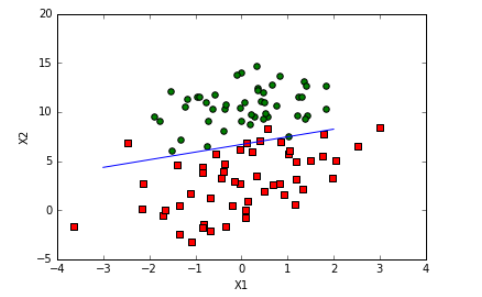• “梯度上升法”的不足在于每次更新回归系数时都要遍历整个数据集，计算复杂度高
因此，产生了“随机梯度上升法”，即每次仅使用1个样本点数据来更新回归系数

### 5-3 随机梯度上升算法

随机梯度上升算法：一次仅用一个样本点来更新回归系数
随机梯度上升伪代码：
每个回归系数初始化为1
对数据集中每个样本：
计算该样本的梯度
返回回归系数

• 和梯度上升法的区别：第一，后者的变量h和error都是向量，而前者则全是数值；第二，前者没有矩阵的转化过程，所有变量的数据类型都是Numpy数组
def stocGradAscent0(dataMatrix, classLabels):
m,n = shape(dataMatrix)  # 取数组的行m， 列n
alpha = 0.01  # 学习率初始化
weights = ones(n)   # 创建 含有 n 个元素的 数组
for i in range(m): # 循环每一行的元素
h = sigmoid(sum(dataMatrix[i]*weights))# sum(dataMatrix[i]*weights)=w0*x0+w1*x1+w2*x2
error = classLabels[i] - h
weights = weights + alpha * error * dataMatrix[i]    # 更新权重
return weights   # 返回权重

#运行代码：
weights = stocGradAscent0(array(dataArr), labelMat)
plotBestFit(weights)
• 结果如图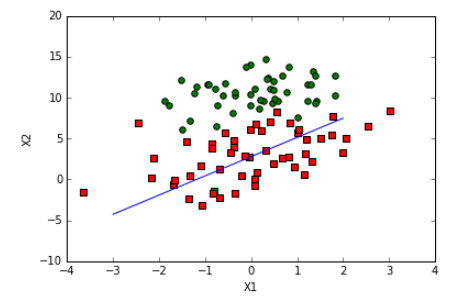• “随机梯度上升法”分错了三分之一，因为原始梯度上升算法（5-1）是在整个数据集上迭代500次得来的，这是不公平，我们对本算法同样进行多次迭代，发现问题如下：
不足在于回归系数存在周期震荡波动。
因此，产生了“改进的随机梯度上升法”，改进之处有两点：
1、动态调整步进因子；
2、随机选取样本来更新回归系数。

### 5-4 改进的随机梯度上升算法

def stocGradAscent1(dataMatrix, classLabels, numIter = 150):#因为由图可知迭代到150次左右就收敛（达到稳定值）
m,n = shape(dataMatrix)   # 取数组（narray）的行，列 m=100， n=3  （narray 和 matrix 长的一样）
weights = ones(n)  # [1. 1. 1.]
for j in range(numIter):  # 循环到 最大循环次数numIter = 150：
# dataIndex = range(m) ### 书中需要更改的地方  tip: TypeError: 'range' object doesn't support item deletion
dataIndex = list(range(m))  # 创建一个0到99， 步长为1 的列表
for i in range(m):   # 循环listIndex
alpha = 4/(1.0+j+i)+0.01#动态调整步进因子
randIndex = int(random.uniform(0, len(dataIndex)))   # 随机选取样本来更新回归系数
h = sigmoid(sum(dataMatrix[randIndex]*weights))   #  1.0/(1+exp(-Z))    Z = dataMatrix * weights
error = classLabels[randIndex] - h
weights = weights + alpha * error * dataMatrix[randIndex]   # 更新权重
del(dataIndex[randIndex])   # 删除用过的 随机数 （避免重复）
return weights   # 返回权重

#运行代码
from numpy import *
weights = stocGradAscent1(array(dataArr), labelMat)
plotBestFit(weights)
• 结果如图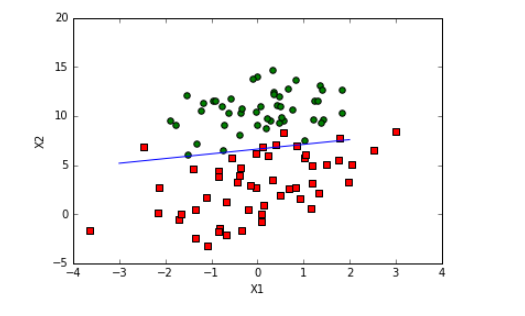### 5-5  示例：从疝气病症预测病马的死亡率

def classifyVector(inX, weights):
prob = sigmoid(sum(inX*weights))  # 输入Z=inX*weights（特征向量*回归系数） 计算Sigmoid值
if prob > 0.5:
return 1.0
else:
return 0.0

def colicTest():
frTrain = open('horseColicTraining.txt')   # 打开训练集
frTest = open('horseColicTest.txt')   # 打开测试集
trainingSet = []   # 训练集列表
trainingLabels = []   # 训练标签列表
#把训练集文本处理放入训练集列表和训练标签列表中
for line in frTrain.readlines():   # 读取训练文本的 每一行
currLine = line.strip().split('\t')   # 除去空格，然后用split方法 转化 成list
lineArr = []   # 定义行数组（处理每行数据）
for i in range(21):  #数据有22列，前21个为特征，最后一个是分类标签
lineArr.append(float(currLine[i]))  # 添加currLine的第i个元素到lineArr
trainingSet.append(lineArr)   # 把 lineArr 作为 子列表 添加到 训练集列表trainingSet[ [],[],[],...]的形式
trainingLabels.append(float(currLine))  #  把 currLine 最后一个元素（是标签） 添加到训练标签trainingLabels
trainWeights = stocGradAscent1(array(trainingSet),trainingLabels,500)   # 迭达500次 求 训练权重
errorCount = 0   #错误计数器
numTestVec = 0.0 #总数计数器
#把测试集文本
for line in frTest.readlines():   # 读取测试文本的每一行
numTestVec += 1.0  # numTestVec == 1.0
currLine = line.strip().split('\t')  # 除去空格，然后用split方法 转化 成list
lineArr = []    # 定义行数组（处理每行数据）
for i in range(21):  # 数据有22列，前21个为特征，最后一个是分类标签
lineArr.append(float(currLine[i]))   # 添加currLine的第i个元素到lineArr
if int(classifyVector(array(lineArr), trainWeights)) != int(currLine):  # 如果分类有错误
errorCount += 1
errorRate = (float(errorCount)/numTestVec)   # 计算错误率
print("the error rate of this test is: %f" % errorRate)
return errorRate
# errorCount,numTestVec 各变量结果  25, 67.0

def multiTest():    # 调用colicTest（）函数10次并求平均值
numTests = 10
errorSum = 0.0
for k in range(numTests):
errorSum += colicTest()
print("after %d iterations the average error rate is: %f" % (numTests, errorSum/float(numTests)))

print(colicTest())
multiTest()



结果 如下：

the error rate of this test is: 0.388060
0.3880597014925373
the error rate of this test is: 0.417910
the error rate of this test is: 0.432836
the error rate of this test is: 0.402985
the error rate of this test is: 0.328358
the error rate of this test is: 0.388060
the error rate of this test is: 0.402985
the error rate of this test is: 0.238806
the error rate of this test is: 0.358209
the error rate of this test is: 0.462687
the error rate of this test is: 0.328358
after 10 iterations the average error rate is: 0.376119

​​​​​

# 参考资料

【1】https://zhuanlan.zhihu.com/p/36670444
【2】https://zhuanlan.zhihu.com/p/36564434
【3】《机器学习》周志华
【4】《机器学习实战》

（仅供个人学习记录）

展开全文机器学习 机器学习实战 分类
• 前言：引入周志华《机器学习》书中对数几率统计中的理论和吴恩达深度学习系列结合自己理解的内容。 应用场景：逻辑回归是一个应用于二分类的算法。其输出标记为，而线性回归模型所产生的预测值是实数，于是将实值z...

前言：引入周志华《机器学习》书中对数几率统计中的理论和吴恩达深度学习系列结合自己理解的内容。

应用场景：逻辑回归是一个应用于二分类的算法。其输出标记为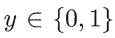，而线性回归模型所产生的预测值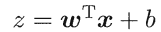是实数，于是将实值z转化为0/1值，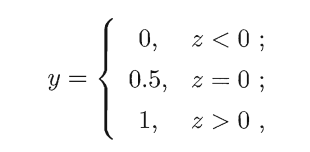如果预测值大于0则标记为1，反之则标记为0.

逻辑回归的假设函数（Hypothesis function）：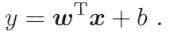•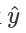值为预测值 更正式的说是表示y等于1的可能性或机会
• w   实际上是特征权重，维度与特征向量相同
• b   表示偏差

这时我们得到是一个关于x的线性函数，但此时对于二分类问题却不是一个非常好的算法，因为如果想让表示实际值y等于1的几率的话，应该是在0~1之间，因为实际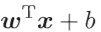的值比1要大的多，或者甚至可能为一个负值。因此我们在逻辑回归中应使用sigmoid函数，将线性函数转化为非线性函数，如下图所示：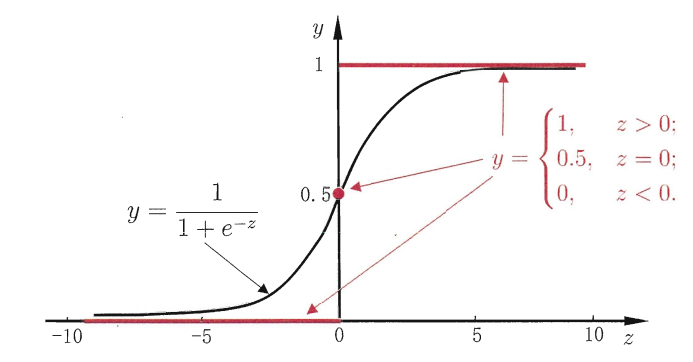sigmoid函数的公式为：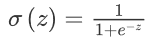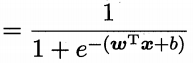，这里z是一个实数，当z的值特别大时，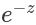趋向于0，而此时sigmoid函数的值趋向于1，反之，当z的值特别小时，sigmoid函数的值趋向于0.，亦可将公式变形为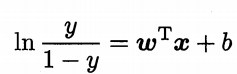，这里将y视为样本x作为正例的可能性，1-y视为作为反例的可能性。则两者的比值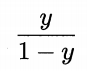，称为几率，反映了x作为正例的相对可能性。所以在实现逻辑回归时，主要任务就说让机器学习参数w和b。

逻辑回归的代价函数（Logistic Regression Cost Function）：

代价函数的作用是训练参数w和参数b，通过给定m个训练样本，我们可以得到w和b，并以此得到预测值。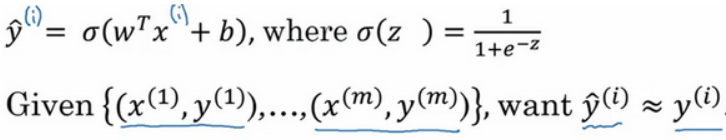上标i的含义是样本的索引。

损失函数（Loss function）：又叫误差函数，用于衡量算法的运行情况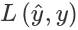，通过L来衡量预测值和真实值得差距，一般情况下我们用到得损失函数是用均方误差来衡量，即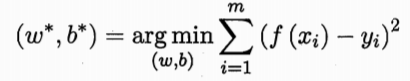，而在逻辑回归中，我们使用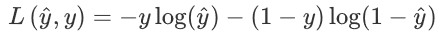来衡量误差值。

• 当y=1时，损失函数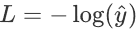，如果想让误差尽可能小，那么就要尽可能大，因为sigmoid函数取值0~1，所以会无限接近于1。
• 当y=1时，损失函数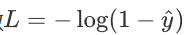，如果想让误差尽可能小，那么就要尽可能小，因为sigmoid函数取值0~1，所以会无限接近于0。

损失函数是在单个函数上定义的，它衡量的是算法在单个训练样本中表现如何，为了衡量算法在全部训练样本上的表现如何，我们需要定义一个算法的代价函数，算法的代价函数是对m个样本的损失函数求和然后除以m: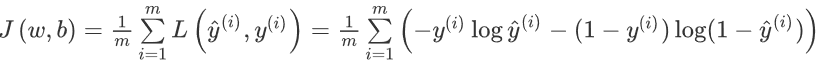损失函数只适用于像这样的单个训练样本，而代价函数是参数的总代价，所以在训练逻辑回归模型时候，我们需要找到合适的w和b，来让代价函数 J的总代价降到最低。

逻辑回归中的梯度下降（Logistic Regression Gradient Descent）：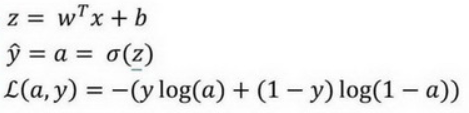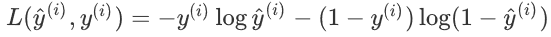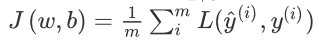w和b的修正量表示如下：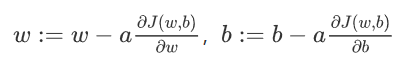假设样本只有两个特征x1和x2，为了计算z，需要输入w1，w2和b，以及特征值x1，x2.

首先我们需要反向计算出代价函数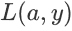关于a的导数,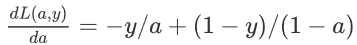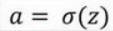,因为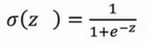所以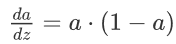，由链式求导法则可知，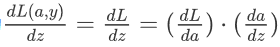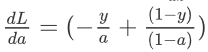所以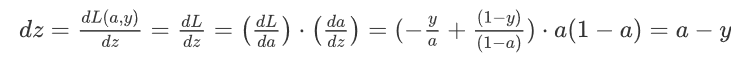又因为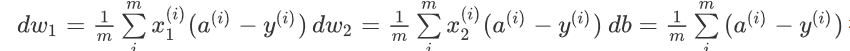dw1 =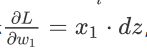,dw2 =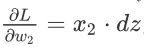,db = dz

所以单个样本的逻辑回归梯度下降要做的就是先用a-y计算出dz，再分别计算出dw1,dw2,db，最后更新w和b的值即完成一次梯度下降

m个样本的梯度下降：

J=0;dw1=0;dw2=0;db=0;

for i = 1 to m

z(i) = wx(i)+b;

a(i) = sigmoid(z(i));

J += -[y(i)log(a(i))+(1-y(i)）log(1-a(i));

dz(i) = a(i)-y(i);

dw1 += x1(i)dz(i);

dw2 += x2(i)dz(i);

db += dz(i);

J/= m;

dw1/= m;

dw2/= m;

db/= m;

w=w-alpha*dw

b=b-alpha*db

将其向量化来简化上述代码

首先来看db，因为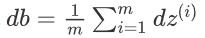，不难看出，db可用1/m*np.sum(dz)来代替，然后来看dw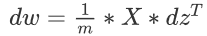,X是一个行向量，因此展开后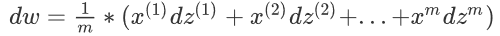则处理后代码可简化为：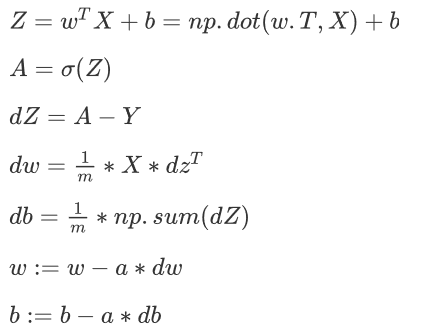代码实际演练 （采取吴恩达深度学习第二周编程作业）：

定义sigmoid函数，返回经过处理后的预测值

def sigmoid(z):
"""
参数：
z  - 任何大小的标量或numpy数组。

返回：
s  -  sigmoid（z）
"""
s = 1 / (1 + np.exp(-z))
return s


计算代价函数及其渐变

def propagate(w, b, X, Y):
"""
实现前向和后向传播的成本函数及其梯度。
参数：
w  - 权重，大小不等的数组（num_px * num_px * 3，1）
b  - 偏差，一个标量
X  - 矩阵类型为（num_px * num_px * 3，训练数量）
Y  - 真正的“标签”矢量（如果非猫则为0，如果是猫则为1），矩阵维度为(1,训练数据数量)

返回：
cost- 逻辑回归的负对数似然成本
dw  - 相对于w的损失梯度，因此与w相同的形状
db  - 相对于b的损失梯度，因此与b的形状相同
"""
m = X.shape

#正向传播
A = sigmoid(np.dot(w.T,X) + b) #计算激活值，请参考公式2。
cost = (- 1 / m) * np.sum(Y * np.log(A) + (1 - Y) * (np.log(1 - A))) #计算成本，请参考公式3和4。

#反向传播
dw = (1 / m) * np.dot(X, (A - Y).T) #请参考视频中的偏导公式。
db = (1 / m) * np.sum(A - Y) #请参考视频中的偏导公式。

#使用断言确保我的数据是正确的
assert(dw.shape == w.shape)
assert(db.dtype == float)
cost = np.squeeze(cost)
assert(cost.shape == ())

#创建一个字典，把dw和db保存起来。
"dw": dw,
"db": db
}
return (grads , cost)


使用渐变下降更新参数w和b

def optimize(w , b , X , Y , num_iterations , learning_rate , print_cost = False):
"""
此函数通过运行梯度下降算法来优化w和b

参数：
w  - 权重，大小不等的数组（num_px * num_px * 3，1）
b  - 偏差，一个标量
X  - 维度为（num_px * num_px * 3，训练数据的数量）的数组。
Y  - 真正的“标签”矢量（如果非猫则为0，如果是猫则为1），矩阵维度为(1,训练数据的数量)
num_iterations  - 优化循环的迭代次数
learning_rate  - 梯度下降更新规则的学习率
print_cost  - 每100步打印一次损失值

返回：
params  - 包含权重w和偏差b的字典
成本 - 优化期间计算的所有成本列表，将用于绘制学习曲线。

提示：
我们需要写下两个步骤并遍历它们：
1）计算当前参数的成本和梯度，使用propagate（）。
2）使用w和b的梯度下降法则更新参数。
"""

costs = []

for i in range(num_iterations):

grads, cost = propagate(w, b, X, Y)

w = w - learning_rate * dw
b = b - learning_rate * db

#记录成本
if i % 100 == 0:
costs.append(cost)
#打印成本数据
if (print_cost) and (i % 100 == 0):
print("迭代的次数: %i ， 误差值： %f" % (i,cost))

params  = {
"w" : w,
"b" : b }
"dw": dw,
"db": db }
return (params , grads , costs)


optimize函数会输出已学习的w和b的值，我们可以使用w和b来预测数据集X的标签。

计算预测值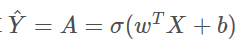将a的值变为0（如果激活值<= 0.5）或者为1（如果激活值> 0.5）

展开全文机器学习
• 数据回归-对数广义幂威布尔回归模型的统计分析.pdf
• 回归分析线性回归Logistic回归对数线性模型PPT学习教案.pptx
• SPSS专题回归分析线性回归Logistic回归对数线性模型PPT教案.pptx
• ## 对数拟合

千次阅读 2021-04-19 01:44:47
各位前辈好，我想请教一下如何在MATLAB中做对数回归下，效果图如下：我自己做了线性回归，代码和图如下，可是如何进行对数回归呢，谢谢for iYrs=2012201559;iy=iYrs;sy=num2str(iy);eval(['load ' Folder Site '_' ...
• 一、线性回归 线性回归（linear regression：试图学得一个线性...二、对数几率回归 在 一 中讲到使用线性模型进行回归学习，若要进行分类任务，应该怎样做呢？ 此时需要借助某些函数，将分类的任务的结果与回归得到的机器学习
• ## stata 应用回归分析

千次阅读 2020-12-12 05:04:39
1. stata软件概述 时间:2020-2-25 窗口介绍 do-flie editor data editor(可编辑) data browser(不可编辑) 功能按钮 graphics ...回归分析 广义线性模型 最大似然估计 广义矩估计 多变量模型 ...
• Logistic回归，也称为Logit模型，用于对二元结果变量进行建模。在Logit模型中，结果的对数概率被建模为预测变量的线性组合。 例子 例1. 假设我们对影响一个政治候选人是否赢得选举的因素感兴趣。结果（因）变量...r语言 逻辑回归
• 对数几率回归函数模型损失函数使用soft-max进行多分类通过分布式系统并行运算使用FTRL加速器进行在线学习 对数几率回归(LR)是机器学习的入门分类器，属于广义线性回归，解决了线性回归不擅长的分类问题，常用于二...softmax FTRL 逻辑回归 LR
• 文章目录回归分析认识回归什么是回归Sklearn中的回归回归模型的应用线性模型（linear model）获得线性模型线性模型的基本形式线性回归目标函数（单变量）目标函数（多变量）python实现数据集划分：线性回归实例逻辑...
• 7个回归分析方法 什么是回归分析回归分析是一种预测性的建模技术，它研究的是因变量（目标）和自变量（预测器）之间的关系。 这种技术通常用于 预测分析、 时间序列模型 以及发现变量之间的...
• ﻿﻿ 如果自变量X和因变量Y的回归方程为对数关系，则为对数回归
• 以航运市场中重要船型-好望角型散货船为研究对象，在分析相关可量化因素与新船价格关系的基础之上，构建双对数线性回归模型分析各因素对新船船价的影响程度，发现对于新造好望角型散货船而言，二手船船价、新造船...
• 机器学习专题(一)梯度下降法实现对数几率回归模型优化 文章目录机器学习专题(一)梯度下降法实现对数几率回归模型优化一、梯度下降算法二、使用步骤1.引入库2.读入数据总结 一、梯度下降算法 示例：pandas 是基于...机器学习 逻辑回归
• 并不是大的量和小的量放在一起大的就要对数，那我必然要问你，为什么不选择开N次方呢？或者把小的求阶乘(当然这种操作可能也不太“自然”)？事实上选择如何处理特征是要有一定的“具象”理由的，常见的两个原因有：...
• 逻辑(logistic)回归, 又称logistic回归分析，是一种广义的线性回归分析模型，常用于数据挖掘，疾病自动诊断，经济预测等领域 1、几率 几率：一个时间发生的概率 / 不发生的概率 2、逻辑 逻辑：指的是logistic ...python 逻辑回归 机器学习 人工智能
• 展开全部取对数作用主要有：缩小数32313133353236313431303231363533e4b893e5b19e31333363386231据的绝对数值，方便计算。例如，每个数据项的值都很大，许多这样的值进行计算可能对超过常用数据类型的取值范围，这时...
• 更多CFA官方教材详解，请关注“边际实验室”。“边际实验室”是一家专注于金融科技...■在估计回归之前，可能需要转换一个或多个回归变量(例如，通过对变量取自然对数)。■回归模型汇集了来自不同样本中不应该被汇集...
• 本文就来梳理下线性回归分析的分析流程，闲话少说，我们开始吧！线性回归回归分析实质上就是研究一个或多个自变量X对一个因变量Y(定量数据)的影响关系情况。当自变量为1个时，是一元线性回归，又称作简单线性回归；...
• 《机器学习：公式推导与代码实践》鲁伟著读书笔记。...在对数几率回归中，我们需要将线性回归模型的预测值转化为0/1值，而不是去逼近真实标签yyy。而取值范围为（0，1），单调可微的Sigmoid函数便是对机器学习 python
• 线性回归其实就是高中讲过的那种回归，但是不同的是线性回归在神经网络这个学科里大多使用梯度下降来计算参数w,bw,bw,b，而高中讲的是使用最小二乘法。 线性回归想解决的问题也是回归问题，公式： y=wx+b y=wx+by=wx...
• 第2章 回归分析 1.回归分析 2.回归分析的实例 3.回归分分析程中的注意事项 4.标准化残差 5.内插法和外插法 6.序列相关 7.直以外的回归方程 第3章 重回归分析 1.重回归分析的定义 2.重回归分析的实例 3.重回归分析...
• 我总是告诉学生，采用自然对数来转换变量的三个原因。记录变量的原因将决定您是要记录独立变量还是因变量或两者。为了清楚起见，我正在谈论采用自然对数。首先，如其他海报所指出的那样，提高模型拟合度。例如，如果...
• 这次和上一次的内容类似，以西瓜书南瓜书为主结合统计学习方法与吴恩达机器学习，先做了一些手写的笔记，后面会精简整理成博客。...